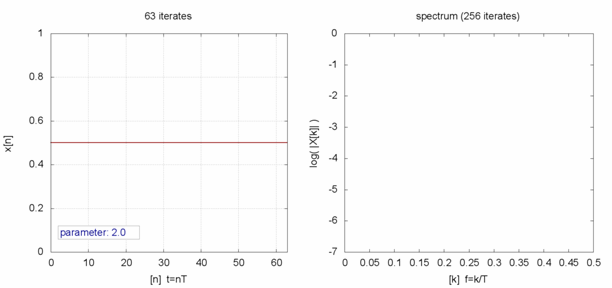#### 有限的人口增长

logistic映射是一种离散递推关系，定义为

$x_{n+1} = rx_{n}(1-x_n)$$f(x)=\frac{1}{1+e^{-x}}$

$1-f(x)=f(-x)$

$\frac{d}{dx}f(x)=\frac{e^{-x}}{(1+e^{-x})^2}$

1838年，皮埃尔·弗朗索瓦·韦吕勒（Pierre François Verhulst）发表了一个用于描述人口增长的模型。在这个模型中，人口的增长率同时与现有人口和可用资源成正比。随着人口的逐渐增长，可用资源将逐渐枯竭，导致增长率逐渐降低，最终人口会趋近于一个定值。
1911年，A. G. McKendrick重新发现了这个模型中的方程，并验证了其对于描述高汤中细菌数量增长的准确性。此后，这个方程又曾数次被重新发现与改进。它的形式很简洁，为

$\frac{dP}{dt}=rP(1-\frac{P}{K})$

$P(t)=\frac{KP_0 e^{rt}}{K+P_0(e^{rt}-1)}$

$\lim_{t\to\infty} P(t)=K$

#### 简单而混沌• $r$=2.9，单周期振荡开始。
• $r$=3.0，双周期振荡开始。
• $r$介于3.4与3.5之间时，四周期振荡。
• $r$介于3.4与3.569946之间时，周期倍增越来越快。
• $r$=3.569946，混沌开始。

• $r/_1$=3.0
• $r/_2$=3.44949
• $r/_3$=3.54409
• $r/_4$=3.564407
• $r/_5$=3.568758
• $r/_6$=3.569692
• $r/_7$=3.569891
• $r/_8$=3.569934

#### 参考文献

https://en.wikipedia.org/wiki/Logistic_map
https://en.wikipedia.org/wiki/Logistic_function
http://rasch.org/rmt/rmt64k.htm

#### 推荐书目

1. 原文为法语，logistique ↩︎

2. 此处指函数中自然对数的底的指数，即$e^x$。 ↩︎